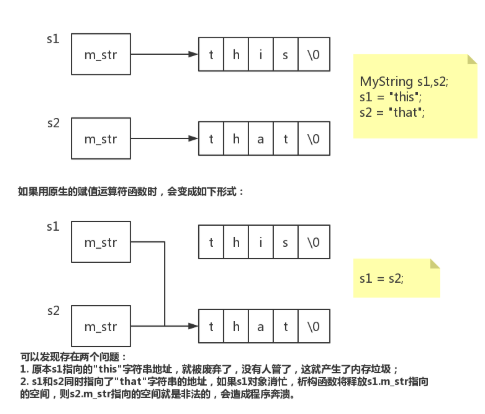# C++ 赋值运算符‘=‘的重载（浅拷贝、深拷贝）

0收藏

01 赋值运算符重载的需求

02 赋值运算符重载的例子

``````class MyString // 字符串类
{
public:
// 构造函数，默认初始化1个字节的字符
MyString ():m_str(new char)
{
m_str = 0;
}

// 析构函数，释放资源
~MyString()
{
delete [] m_str;
}

const char* c_str()
{
return m_str;
}

// 赋值运算符重载函数
// 重载=号使得 obj = "Hello" 能够成立
MyString & operator= (const char *s)
{
// 释放旧字符串资源
delete [] m_str;

// 生成新字符串的空间大小，长度多+1的目的是存放\0
m_str = new char[strlen(s) +1 ];

// 拷贝新字符串的内容
strcpy(m_str, s);

// 返回该对象
return *this;
}

private:
char * m_str; // 字符串指针
};

int main() {

MyString s;

s = "Hello~"; // 等价于s.operator=("Hello~");
std::cout << s.c_str()  << std::endl;

// MyString s2 = "Hello!"; // 这条语句要是不注释就会编译报错

s = "Hi~"; // 等价于s.operator=("Hi~");
std::cout << s.c_str()  << std::endl;

return 0;
}``````

``````Hello~
Hi~``````

03 浅拷贝和深拷贝

``````MyString s1,s2;
s1 = "this"; // 调用重载的赋值语句
s2 = "that"; // 调用重载的赋值语句
s1 = s2; // 如何实现这个？？``````

s1 = s2;语句目的希望是s1对象放的字符串和s2对象放的字符串象要一样，由于 = 号两边的类似都是对象，编译器会用原生的赋值运算符函数，但是这个原生的赋值运算符函数对于有指针成员变量的对象来说，是非常危险的！``````MyString & operator=(const MyString & s)
{
// 释放旧字符串资源
delete [] m_str;

// 生成新字符串的空间大小，长度多+1的目的是存放\0
m_str = new char[strlen(s.m_str) +1 ];

// 拷贝新字符串的内容
strcpy(m_str, s.m_str);

// 返回该对象
return *this;
}``````

``````MyString s;
s = "Hello";
s = s; // 是否会有问题？``````

s = s;等价于s.operator=(s)，由于s和s是相同的对象，那么就没必要完全执行重载的赋值 = 的函数了，我们再加个判断，当左右两边是相同对象时，就直接返回该对象就好：

``````MyString & operator=(const MyString & s)
{
// 当左右两边是相同对象时，就直接返回该对象就
if(this == &s)
return *this;

delete [] m_str;
m_str = new char[strlen(s.m_str) +1 ];
strcpy(m_str, s.m_str);
return *this;
}``````

• void 好不好？
• MyString 好不好？
• 为什么是MyString &？

• a = b = c; 这个赋值语句的顺序是先b = c，然后在a = (b = c)。如果返回的void类型，那么a = (void)显然是不成立的；
• (a = b) = c; 这个赋值语句会修改a的值，如果返回的类型是MyString对象，那么就无法修改a的值了。

• a.operator=(b.operator=(c));
• (a.operator=(b)).operator=(c);

04 复制（拷贝）构造函数

``````MyString s;
s = "Hello";
MyString s1(s); // 要考虑这种情况，那就要重载复制（拷贝）构造函数``````

``````MyString (const MyString &s)
{
m_str = new char[strlen(s.m_str) + 1];
strcpy(m_str, s.m_str);
}``````

05 小结

``````class MyString // 字符串类
{
public:
// 构造函数，默认初始化1个字节的字符
MyString ():m_str(new char)
{
m_str = 0;
}

// 复制（拷贝）构造函数
MyString (const MyString &s)
{
m_str = new char[strlen(s.m_str) + 1];
strcpy(m_str, s.m_str);
}

// 析构函数，释放资源
~MyString()
{
delete [] m_str;
}

const char* c_str()
{
return m_str;
}

// 赋值运算符重载函数
// 重载=号使得 obj = "Hello" 能够成立
MyString & operator= (const char *s)
{
// 释放旧字符串资源
delete [] m_str;

// 生成新字符串的空间大小，长度多+1的目的是存放\0
m_str = new char[strlen(s) +1 ];

// 拷贝新字符串的内容
strcpy(m_str, s);

// 返回该对象
return *this;
}

// 赋值运算符重载函数
// 重载=号使得 obj1 = obj2 能够成立
MyString & operator=(const MyString & s)
{
// 当左右两边是相同对象时，就直接返回该对象就
if(this == &s)
return *this;

delete [] m_str;
m_str = new char[strlen(s.m_str) +1 ];
strcpy(m_str, s.m_str);
return *this;
}

private:
char * m_str; // 字符串指针
};

int main()
{

MyString s1,s2;

s1 = "Hello~"; // 等价于s1.operator=("Hello~");
std::cout << s1.c_str()  << std::endl;

s2 = "Hi~"; // 等价于s2.operator=("Hi~");
std::cout << s2.c_str()  << std::endl;

s1 = s2;   // 等价于s1.operator=(s2);
std::cout << s1.c_str()  << std::endl;

MyString s3(s1);  // 复制构造函数
std::cout << s3.c_str()  << std::endl;

return 0;
}``````

``````Hello~
Hi~
Hi~
Hi~``````

1帖子
视频
声望
粉丝
社区精华内容